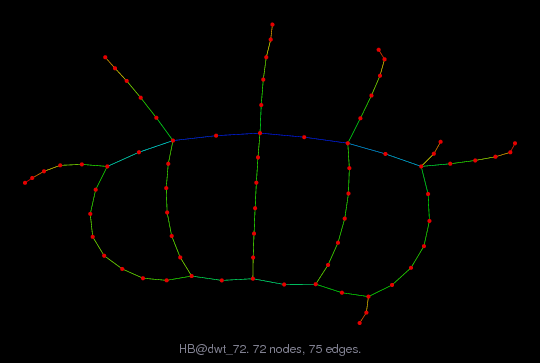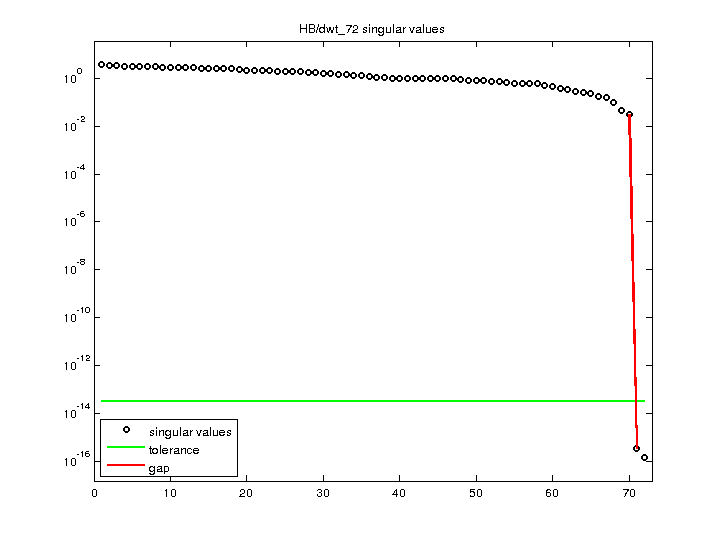Matrix: HB/dwt_72

Description: SYMMETRIC CONNECTION TABLE FROM DTNSRDC, WASHINGTON(undirected graph drawing)• Matrix group: HB
• download as a MATLAB mat-file, file size: 862 bytes. Use UFget(133) or UFget('HB/dwt_72') in MATLAB.

 Matrix properties number of rows 72 number of columns 72 nonzeros 222 structural full rank? yes structural rank 72 # of blocks from dmperm 1 # strongly connected comp. 1 explicit zero entries 0 nonzero pattern symmetry symmetric numeric value symmetry symmetric type binary structure symmetric Cholesky candidate? yes positive definite? no

 author G. Everstine, D. Taylor editor I. Duff, R. Grimes, J. Lewis date 1980 kind structural problem 2D/3D problem? yes

 Ordering statistics: result nnz(chol(P*(A+A'+s*I)*P')) with AMD 184 Cholesky flop count 4.9e+02 nnz(L+U), no partial pivoting, with AMD 296 nnz(V) for QR, upper bound nnz(L) for LU, with COLAMD 192 nnz(R) for QR, upper bound nnz(U) for LU, with COLAMD 321

 SVD-based statistics: norm(A) 3.49232 min(svd(A)) 1.60781e-16 cond(A) 2.1721e+16 rank(A) 70 sprank(A)-rank(A) 2 null space dimension 2 full numerical rank? no singular value gap 8.30154e+13

 singular values (MAT file): click here SVD method used: s = svd (full (A)) ; status: ok